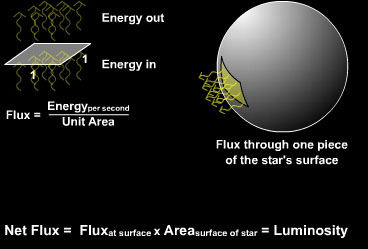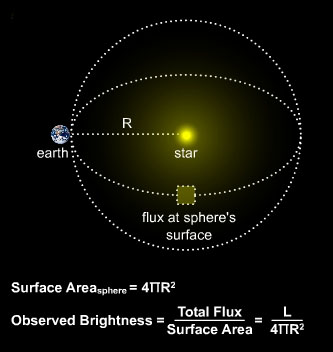### LuminosityJust as we can measure the energy output of a power plant, so can we for a star. Every second, a certain amount of energy (mostly in the form of light) is flowing radially outward from a star.

If we sum the energy flowing out of a star per second, we get the star's luminosity, L. Essentially, we are adding up the "flux", or flow of energy through a unit area over the entire surface of the star. So, a star's luminosity is equivalent to the star's "total surface flux", which is equal to the flux at the surface of the star, multiplied by the surface area of the star.

### Observed BrightnessWe don't necessarily have to measure flux at the surface of a star. We can measure the flux from a star at any point in space.

Imagine a giant mathematical sphere (with a star at its center) whose radius (R) is the star's distance to an observer on Earth. The flux at the surface of the sphere is what we call the observed brightness of the star.

Observed brightness, b is defined as the energy passing through a unit area at the observer every second. To find b, we divide the star's net surface flux (luminosity) by the mathematical sphere's surface area. As one can see, the flux at the star's surface is much greater than the flux at distance R.